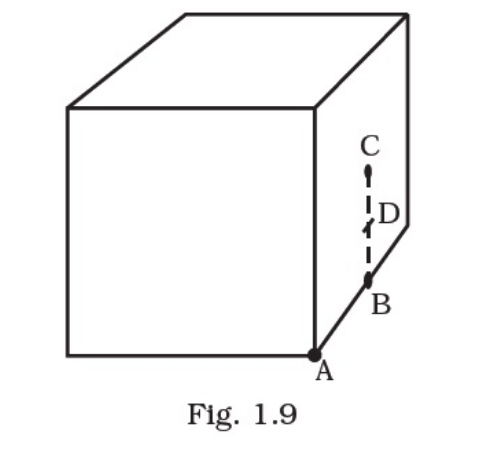# What will be the total flux through

Question:

What will be the total flux through the faces of the cube (Fig. 1.9) with side of length a if a charge is placed at

(a) A: a corner of the cube.

(b) B: mid-point of an edge of the cube.

(c) C: centre of a face of the cube.

(d) D: mid-point of B and C.Solution:

(a) When charge is placed at corner A of the cube then only 1/8th portion of the charge lies incised the Gaussian surface. So, total flux through the faces of the given cube = q/8εo.

(b) When charge is placed at point B of the cube then only 1/4th portion of the charge lies incised the Gaussian surface. So, total flux through the faces of the given cube = q/8εo.

(c) When charge is placed at point C of the cube then only 1/2th portion of the charge lies incised the Gaussian surface. So, total flux through the faces of the given cube = q/2εo.

(d) When charge is placed at point D of the cube then only 1/2th portion of the charge lies incised the Gaussian surface. So, total flux through the faces of the given cube = q/2εo.